### Home > CCA > Chapter 9 > Lesson 9.1.1 > Problem9-7

9-7.
1. For each of the following equations, indicate whether its graph would be a line or a parabola. Homework Help ✎

1. 5x + 2y = 7

2. y = 3x2

3. y = 3

4. 4x2 + 3x = 7 + y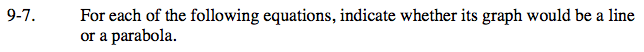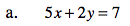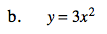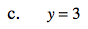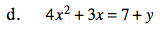If the graph of an equation is a line, it can be written in y = mx + b form.
If the graph of an equation is a parabola, it can be written in y = ax2 + bx + c form.